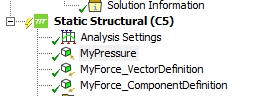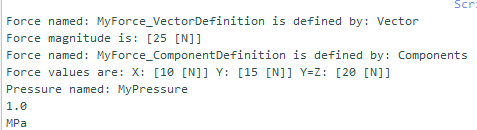# Extract information on boundary conditions in MechanicalMember, Moderator, Employee Posts: 596Is there a script to get boundary conditions defined in Mechanical, know the values of the applied forces and the unit system that is used to define these conditions ?

Tagged:

•Member, Moderator, Employee Posts: 596edited September 8

Here is an example.
The dummy model used is:This scripts prints out the requested information:

``` # Get analysis
analysis = ExtAPI.DataModel.Project.Model.Analyses
# Grab different types of boundary conditions
forces = analysis.GetChildren(DataModelObjectCategory.Force, True)
pressures= analysis.GetChildren(DataModelObjectCategory.Pressure, True)
# Extract and print info on forces
for f in forces:
print("Force named: " + f.Name + " is defined by: " + str(f.DefineBy))
print('Force values are: ' + 'X: ' + str(f.XComponent) + ' Y: '+ str(f.YComponent) + ' Y=Z: '+ str(f.ZComponent))
print('Force magnitude is: ' + str(f.Magnitude))

# Extract and print info on pressures
for p in pressures:
print("Pressure named: " + p.Name)
print('Pressure magnitude is: ' + str(p.Magnitude))
```

There are also some methods that can be used to separate the value itself from the unit system.
For example:

```for p in pressures:
print("Pressure named: " + p.Name)